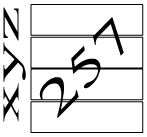# Java Variables, Arrays and Operators

In this lesson the point of discussion would be Variable, Array and Operators.

## Variables in Java

A variable is actually a name of memory location. It can be of any data type. When you declare the variable then you mention its name and the type of data it should accept (store).

```
type variablename;
```

For example,

```
int xyz;
```

This statement has declared a variable whose data will be of int type. As int type takes 4 bytes of memory so this variable will cover 4 bytes.Now if I assign a value to this variable i.e. xyz = 257; then that value will be stored on 4 bytes of memory which were marked as xyz.### Variable Names

Keep in mind the following points in mind for the name of a variable:
1. The variable names can be alphanumeric means combination of alphabets and digits but the name should always start from an alphabet.
2. There should be no space between a variable name i.e. ab xy is a wrong variable name.
3. It is better if the variable name shows its purpose i.e. max is a good name for a variable if you are storing maximum value in it.

## Arrays in Java

Array is basically a collection of similar type data. To fulfill the need of many variables of same data type, array is being used.

```
type  arrayname[length];
```

An array index starts from 0 in Java. So the index would be 0 to {length-1}.
For example, if you want to store the record of roll nos. of 25 students, you will declare an int type array.

```
int  studentRollno;
```

The length of above declared array is 25. So index would be 0 to 24. The assignment to individual element of array would be as follows:

```
studentRollno = 124;
studentRollno = 156;
studentRollno = 183;
.
.
studentRollno = 456;
studentRollno = 458;
```

Note that array consists of contiguous memory locations.
An array can be multi-dimensional. So a 2- dimensional array would be like

```
int arr;
```

This array arr would have following elements arr, arr, arr, arr, arr, and arr.

## Operators in Java

There are many types of operators in Java but the most important are only four i.e. Arithmetic operators, Relational Operators, Assignment Operator, Short-circuit Operator.

### Arithmetic Operators

These operators are used to perform arithmetic operations.

```
Operator         Description
-	        For subtraction
*	        For multiplication
/	        For division
%	        For finding remainder
+=	        First add then assign it
-=	        First subtract then assign it
*=	        First multiply then assign it
/=	        First divide then assign it
%=	        First find remainder then assign it
```

### Relational Operators

These operators are used in conditions for comparison purpose.

```
Operator     Description
==	    To check equality
!=	    To check inequality
>	    Greater than
<	    Less than
>=	    Greater than or equal to
<=	    Less than or equal to
```

### Assignment Operator

This is the most commonly used operator in Java. Assignment operator is denoted by “=” sign (equal sign). For example,

```
int num;
num = 10;
```

Now 10 is assigned to variable num.

### Short-circuit Operators

There are three short-circuit operators.

```
Operator     Description
&&	    AND-true if both are true
||	    OR-true if anyone is true
!	    Negation-make true to false and false to true
```

Operators Example

```
public class MyOperators
{
public static void main(String arg[])
{
int x1;
int value;
x1 = 12;
value = 6;
int newVal = x1/value;
int checkVal = 30-(value*2);
if((checkVal>newVal) && (checkVal>=0))
{
System.out.println(“checkVal is greater.”);
}
else
{
System.out.println(“newVal is greater.”);
}
}
}
```

Save this program as MyOperators.java then compile and run it.
Note that program starts executing in the main(String arg[]) method of the class.
First of all some variables have been declared and some assignments have been done.
The statement int newVal = x1/value; initializes (declares and performs assignment at the same time) the variable newVal to the result of arithmetic operation division. As 12/6 results in 2 so this output will be assigned to newly created variable newVal.
On next statement int checkVal = 30-(value*2); first value i.e. 6 is multiplied with 2 that results in 12. Now 12 will be subtracted from 30 would result in 18. So finally 18 would be assigned to the variable checkVal.
At if statement, condition is being checked that either checkVal is greater than newVal or not. It further checks that checkVal should be a positive number.

3 out of 5 based on 13 user ratings